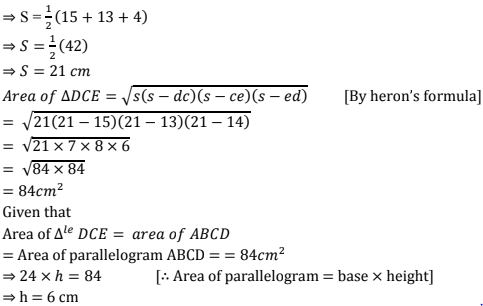# Chapter 12 Heron's Formula RD Sharma Solutions Exercise 12.2 Class 9 Maths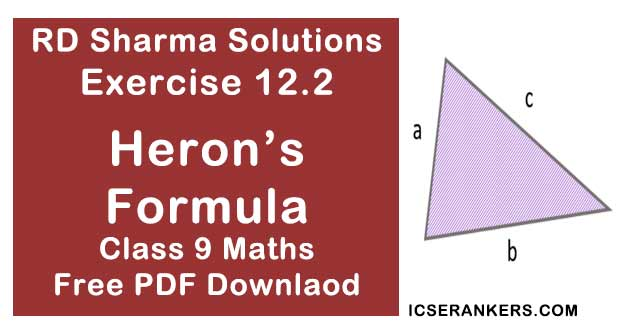Chapter Name RD Sharma Chapter 12 Heron's Formula Exercise 12.2 Book Name RD Sharma Mathematics for Class 10 Other Exercises Exercise 12.1 Related Study NCERT Solutions for Class 10 Maths

### Exercise 12.2 Solutions

1. Find the area of a quadrilateral ABCD is which AB = 3cm, BC = 4 cm, CD = 4cm, DA = 5 cm and AC = 5 cm.

Solution

For ΔPQR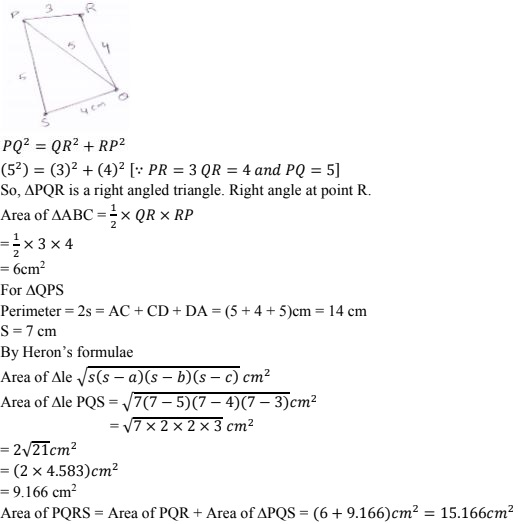2. The sides of a quadrangular field, taken in order are  26 m, 27 m, 7 m are 24 m respectively.  The angle contained by the last two sides is a right angle. Find its area.
Solution
The sides of a quadrilateral field taken order as AB  = 26m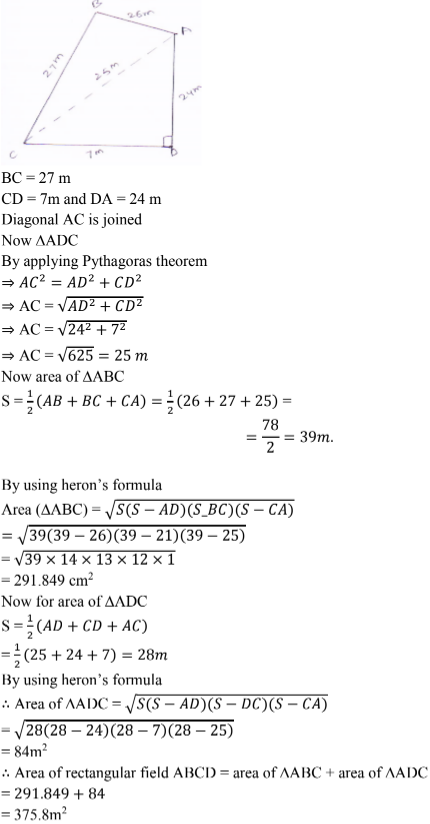3. The sides of a quadrilateral, taken in order are 5, 12,14 and 15 meters respectively, and the angle contained by the first two sides is a right angle. Find its area.
Solution
Given that sides of quadrilateral are AB = 5 m, BC = 12 m, CD = 14 m and DA = 15 m
AB = 5m, BC = 12m, CD = 14 m and DA = 15 m
Join AC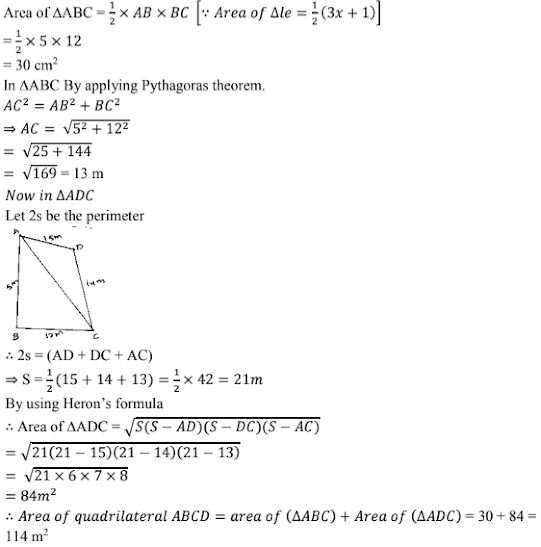4. A park, in the shape of a quadrilateral ABCD has ∠C = 900, AB = 9m, BC = 12 m, CD = 5 m and AD = 8m . How much area does it occupy ?
Solution
Given sides of a quadrilaterals are AB = 9, BC = 12, CD = 05, DA = 08
Let us joint BD
In ∆BCD applying Pythagoras theorem.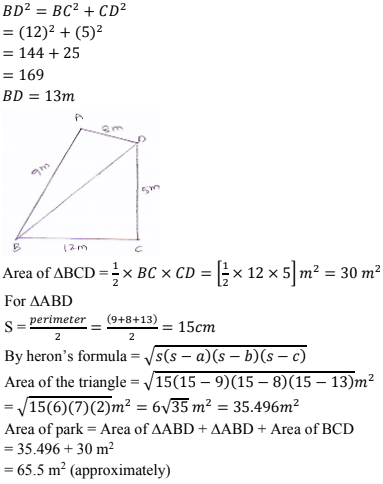5. Two parallel side of a trapezium are 60 cm and 77 cm and other sides are 25 cm and 26 cm.
Find the area of the trapezium.
Solution
Given that two parallel sides of trapezium are AB = 77 and CD = 60 cm
Other sides are BC = 26 m and AD = 25 cm.
Join AE and CF
Now, DE ⊥ AB and CF ⊥ AB
∴ DC = EF = 60 cm
Let AE = x
⇒ BF = 77 – 60 – x = 17 – x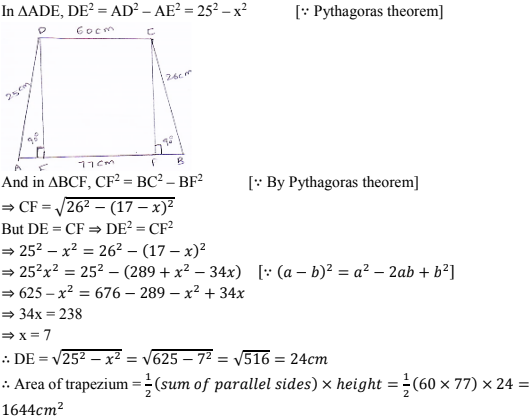6. Find the area of a rhombus whose perimeter is 80 m and one of whose diagonal is 24 m.
Solution
Given that,
Perimeter of rhombus = 80m
Perimeter of rhombus = 4 × side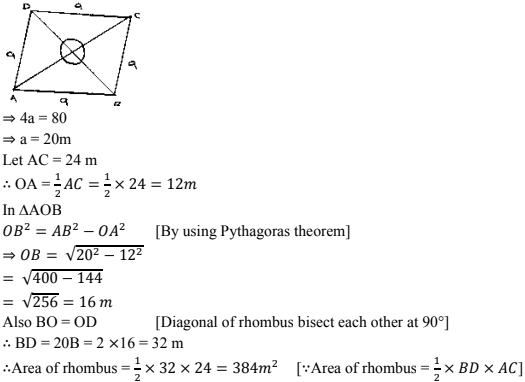7. A rhombus sheet, whose perimeter is 32 m and whose one diagonal is 10 m long, is painted
on both sides at the rate of Rs 5 per m2. Find the cost of painting.
Solution
Given that,
Perimeter of a rhombus = 32 m
We know that,
Perimeter of rhombus = 4 × side
⇒ 49 = 32m
⇒ a = 8 m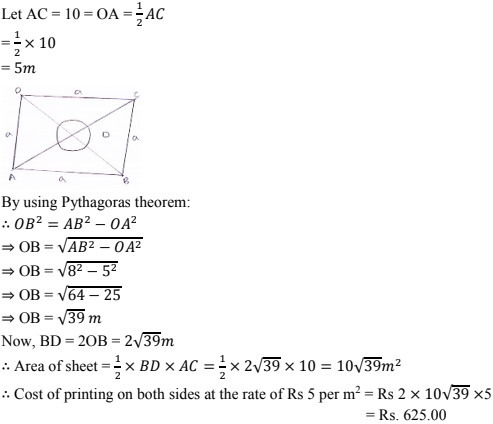8. Find the area of a quadrilateral ABCD in which AD = 24 ∠BAD = 90° and BCD forms
an equilateral triangle whose each side is equal to 26 cm. (Take √3 = 1.73)
Solution
BCD is equilateral triangle and sides BC = CD = BD = 26 cm
In ∆BAD By using Pythagoras theorem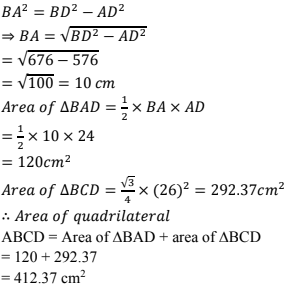9. Find the area of a quadrilateral ABCD in which AB = 42 cm, BC = 21 cm, CD = 29 cm,
DA =34 cm and diagonal BD =20 cm.
Solution
Given that
Sides of a quadrilateral are AB = 42 cm, BC = 21 cm, CD = 29 cm
DA = 34 cm and diagonal BD = 20 cm
Now, area of ∆ABD
Perimeter of ∆ABD
We know that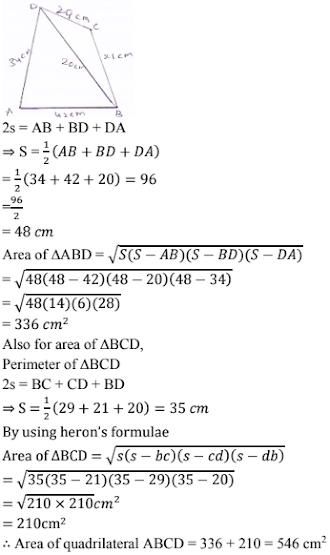10. Find the perimeter and area of the quadrilateral ABCD in which AB = 17 cm, AD =9 cm,
CD = l2cm, ∠ACB = 90° and AC=l5cm.
Solution
The sides of a quadrilateral ABCD in which AB = 17 cm, AD = 9 cm, CD = 12 cm, ∠ACB
= 90° and AC = 15 cm
Here, By using Pythagoras theorem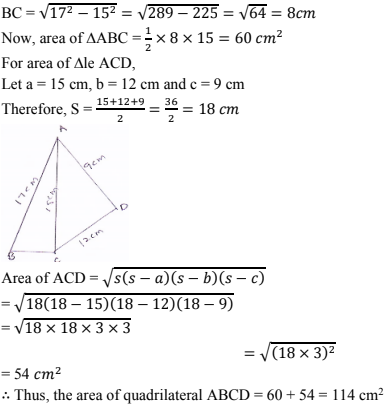11. The adjacent sides of a parallelogram ABCD measure 34 cm and 20 cm, and the diagonal
AC measures 42 cm. Find the area of the parallelogram.
Solution
Given that adjacent sides of a parallelogram ABCD measure 34 cm and 20 cm, and the
diagonal AC measures 42 cm.
Area of parallelogram = Area of ∆ADC + area of ∆ABC
[∵ Diagonal of a parallelogram divides into two congruent triangles]
= 2 × [Area of ∆ABC]
Now for Area of ∆ABC
Let 2s = AB + BC + CA [∵ Perimeter of ∆ABC]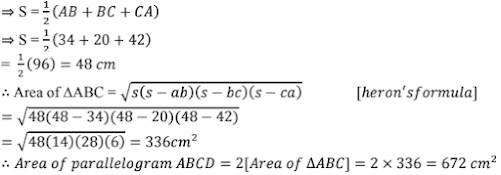12. Find the area of the blades of the magnetic compass shown in Fig.. 12.27.(Take √11 = 3.32).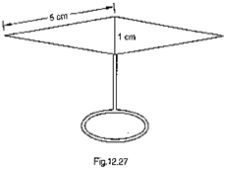Solution
Area of the blades of magnetic compass = Area of ∆ADB + Area of ∆CDB13. A hand fan is made by stitching lo equal size triangular strips of two different types of
paper as shown in Fig. 12.28. The dimensions of equal strips are 25 cm, 25 cm and 14 cm.
Find the area of each type of paper needed to make the hand fan.Solution
Given that the sides of ∆AOB are
AO = 24 cm
OB = 25 cm
BA = 14 cm
Area of each equal strips = Area of ∆le AOB
Now, for area of ∆AOB
Perimeter of ∆AOB
Let 2s = AO + OB + BA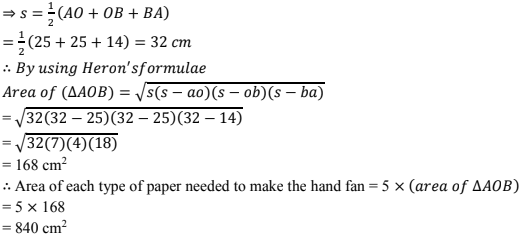14. A triangle and a parallelogram have the same base and the same area. If the sides of the
triangle are 13 cm, 14 cm and 15 cm and the parallelogram stands on the base 14 cm, find
the height of the parallelogram.
Solution
The sides of a triangle DCE are
DC = 15 cm, CE = 13 cm, ED = 14 cm
Let h be the height of parallelogram ABCD
Given,
Perimeter of ∆DCE
2s = DC + CE + ED